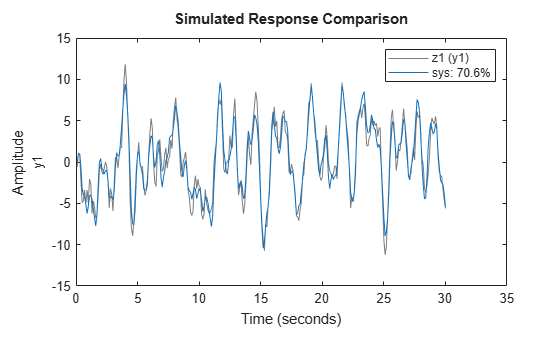Documentation

# goodnessOfFit

Goodness of fit between test and reference data

## Syntax

```fit = goodnessOfFit(x,xref,cost_func) ```

## Description

`fit = goodnessOfFit(x,xref,cost_func)` returns the goodness of fit between the data, `x`, and the reference, `xref` using a cost function specified by `cost_func`.

## Input Arguments

 `x` Test data. `x` is an `Ns`-by-`N` matrix, where `Ns` is the number of samples and `N` is the number of channels. `x` can also be a cell array of multiple test data sets. `x` must not contain any `NaN` or `Inf` values. `xref` Reference data. `xref` must be of the same size as `x`. `xref` can also be a cell array of multiple reference sets. In this case, each individual reference set must be of the same size as the corresponding test data set. `xref` must not contain any NaN or Inf values. `cost_func` Cost function to determine goodness of fit. `cost_func` is specified as one of the following values: `'MSE'` — Mean square error:`$fit=\frac{{‖x-xref‖}^{2}}{Ns}$`where, Ns is the number of samples, and ‖ indicates the 2-norm of a vector. `fit` is a scalar value.`'NRMSE'` — Normalized root mean square error:`$fit\left(i\right)=1-\frac{‖xref\left(:,i\right)-x\left(:,i\right)‖}{‖xref\left(:,i\right)-mean\left(xref\left(:,i\right)\right)‖}$`where, ‖ indicates the 2-norm of a vector. `fit` is a row vector of length `N` and ```i = 1,...,N```, where `N` is the number of channels.NRMSE costs vary between `-Inf` (bad fit) to 1 (perfect fit). If the cost function is equal to zero, then `x` is no better than a straight line at matching `xref`.`'NMSE'` — Normalized mean square error:`$fit\left(i\right)=1-\frac{{‖xref\left(:,i\right)-x\left(:,i\right)‖}^{2}}{{‖xref\left(:,i\right)-mean\left(xref\left(:,i\right)\right)‖}^{2}}$`where, ‖ indicates the 2-norm of a vector. `fit` is a row vector of length `N` and ```i = 1,...,N```, where `N` is the number of channels.NMSE costs vary between `-Inf` (bad fit) to 1 (perfect fit). If the cost function is equal to zero, then `x` is no better than a straight line at matching `xref`.

## Output Arguments

 `fit` Goodness of fit between test and reference data. For a single test data set and reference pair, `fit` is returned as a: Scalar if `cost_func` is `MSE`.Row vector of length `N` if `cost_func` is `NRMSE` or `NMSE`. `N` is the number of channels. If `x` and/or `xref` are cell arrays, then `fit` is an array containing the goodness of fit values for each test data and reference pair.

## Examples

collapse all

Obtain the measured output.

```load iddata1 z1 yref = z1.y;```

`z1` is an `iddata` object containing measured input/output data. `z1.y` is the measured output.

Obtain the estimated output.

```sys = tfest(z1,2); y_sim = sim(sys,z1(:,[],:));```

`sys` is a second-order transfer function estimated using the measured input/output data. `y` is the output estimated using `sys` and the measured input.

Calculate the goodness of the fit between the measured and estimated outputs.

```cost_func = 'NRMSE'; y = y_sim.y; fit = goodnessOfFit(y,yref,cost_func);```

The goodness of fit is calculated using the normalized root mean square error as the cost function.

Alternatively, you can use `compare` to calculate the goodness of fit:

```opt = compareOptions('InitialCondition','z'); compare(z1,sys,opt);```